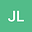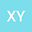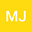The Lie symmetry analysis, exact solutions and conservation laws for variable coefficients Broer-Kaup-Kupershmit equation
•••• +1Xinying Yan
Liao Cheng University
Author ProfileMeng Jin
Liao Cheng University
Author ProfileXiangpeng Xin
Liao Cheng University
Author Profile## Abstract

In this article, the (2+1) - dimensional variable coefficients Broer-Kaup-Kupershmit equation is studied for the first time by Lie symmetry analysis. The derivation process of generating elements of vcBKK equation is given systematically, and the optimal system of the one-dimensional subalgebras is determined. Furthermore vcBKK equation is reduced based on the optimal system, and then the reduced equations are solved with the help of the (G’/G)-expansion method. The images of various kinds of exact solutions are drawn. Finally, according to the conservation theorem, the conservation laws of vcBKK equation is constructed.